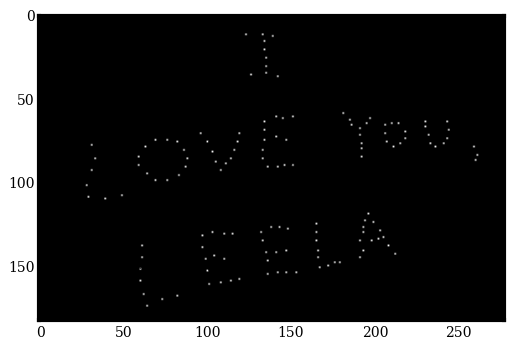This code is meant to simulate the orbital evolution of Leela's constellation from Futurama episode "Time Keeps on Slippin'".

Keep in mind the orbit simulator REBOUND is really designed to evolve well-defined Keplerian orbits, which the stars in Leela's constellation must definitely do not occupy.

In :
import rebound
import numpy as np
import ipywidgets


First, grab image of Leela's constellation. I then made a png in gimp with one pixel defining each stellar center. That's what displayed below.

In :
%matplotlib inline

#From http://stackoverflow.com/questions/36372068/how-to-get-x-y-coordinates-using-python
#
#Took picture of Leela's constellation from google

import cv2
import matplotlib.pyplot as plt

filename = 'Leelas_constellation.png'
# plt.imshow(img)

img=cv2.cvtColor(img,cv2.COLOR_BGR2GRAY) #converting to grayscale
img=img.astype(np.uint8)
cp_img = img.copy()
img[cp_img == 255] = 0
img[cp_img == 0] = 1
plt.imshow(img, cmap='gray')

#get all non zero values
img = np.flipud(img)
coord=cv2.findNonZero(img)Then create REBOUND simulation.

In :
sim = rebound.Simulation()
sim.integrator = "ias15"
sim.collision = "direct"
sim.collision_resolve = "merge"

mass = 1.

for i in range(len(coord)):

sim.move_to_com()

In :
sim.getWidget(size=(400,400))

In [ ]:
import time

time.sleep(10)

for t in np.arange(0., 40., 0.1):
sim.integrate(t)
time.sleep(0.1)


Finally, I did a quicktime screencast and then converted that into a gif via the following command:

ffmpeg -i Leela\'s\ Constellation\ animation.mov -pix_fmt rgb24 -r 10 -f gif - | gifsicle --optimize=3 --delay=3 > Leela\'s\ Constellation\ animation.gif